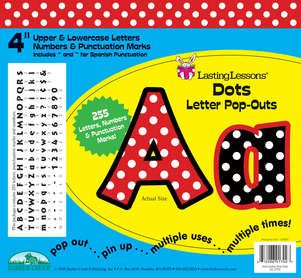•# Dots 4" Letter Pop-Outs

\$9.99

4" Pop-Out Letters
255 Pieces
Set Includes Uppercase and Lowercase Letters, Numbers, Punctuation, and Spanish Punctuation
All Ages! All Grades!

Our Dots Pop-Outs are a cute and classy mix of our Red & White Dot and Black & White Dot designs! Your next decorating project will "pop" with these colorful and classy 4" letters from Barker Creek! Super chic and easy to read, they are perfect for your next project, presentation, report, poster, or display.

Here's what you get: A = 3, B = 2, C = 3, D = 3, E = 3, F = 3, G = 3, H = 3, I = 3, J = 2, K = 2, L = 3, M = 3, N = 3, O = 3, P = 3, Q = 2, R = 3, S = 3, T = 3, U = 3, V = 2, W = 3, X = 2, Y = 3, Z = 2, a = 6, b = 3, c = 4, d = 4, e = 6, f = 4, g = 4, h = 4, i = 6, j = 3, k = 3, l = 4, m = 4, n = 4, o = 4, p = 4, q = 3, r = 4, s = 4, t = 4, u = 4, v = 3, w = 4, x = 3, y = 4, z = 3, ? = 2, ! = 2, ¢=2, \$ = 2, & = 2, . = 18, , = 17, - = 3, " = 4, ~ = 4, ' = 5, and 2 each of numbers 0-9